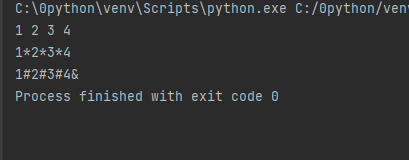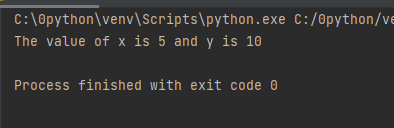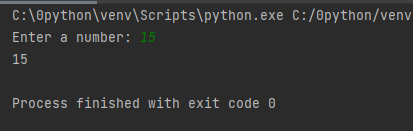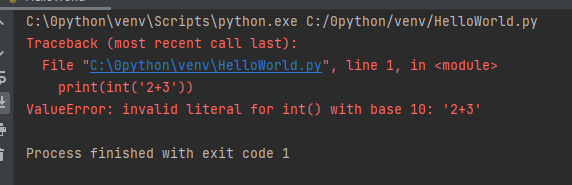# Python 输入、输出和导入

Python 提供了许多内置函数，我们可以在 Python 编程中随时使用这些函数。

input() 和 print() 等一些函数分别广泛用于标准输入和输出操作。让我们先看看输出部分。

## 1. Python 输出内置函数print()

print() 函数将数据输出到标准输出设备（屏幕），也可以将数据输出到文件中，输出到文件中将在后面讨论。

``print('This sentence is output to the screen')``

``This sentence is output to the screen``

``````a = 5
print('The value of a is', a)``````

``The value of a is 5``

print() 函数的实际语法是：

``print(*objects, sep=' ', end='\n', file=sys.stdout, flush=False)``

sep 分隔符用于值之间。它默认为空格字符。

file=是在什么地方打印出对象的值，其默认值为 sys.stdout（屏幕）输出。

``````print(1, 2, 3, 4)
print(1, 2, 3, 4, sep='*')
print(1, 2, 3, 4, sep='#', end='&')``````### 输出格式

``````x = 5; y = 10
print('The value of x is {} and y is {}'.format(x,y))````````````print('I love {0} and {1}'.format('bread','butter'))

``````I love bread and butter

``print('Hello {name}, {greeting}'.format(greeting = 'Goodmorning', name = 'John'))``

``Hello John, Goodmorning``

``````x = 12.3456789
print('The value of x is %3.2f' %x)``````
``The value of x is 12.35``
``````x = 12.3456789
print('The value of x is %3.4f' %x)``````

``The value of x is 12.3457``

## 2.Python 输入

input() 的语法是：

``input([prompt])``

``````num = input('Enter a number: ')
print(num)````````````num = input('Enter a number: ')
print(num)
print(int(num))
print(float(num))``````
``````Enter a number: 15
15
15
15.0``````

``print(eval('2+3'))``

``5``

``print(int('2+3'))``## 3. Python 导入

``import math``

``````import math
print(math.pi)``````

``3.141592653589793``

``````from math import pi
print(pi)``````

``3.141592653589793``

``````import sys
print(sys.path)``````

``['C:\\0python\\venv', 'C:\\0python', 'C:\\Users\\steve\\AppData\\Local\\Programs\\Python\\Python310\\python310.zip', 'C:\\Users\\steve\\AppData\\Local\\Programs\\Python\\Python310\\DLLs', 'C:\\Users\\steve\\AppData\\Local\\Programs\\Python\\Python310\\lib', 'C:\\Users\\steve\\AppData\\Local\\Programs\\Python\\Python310', 'C:\\0python\\venv', 'C:\\0python\\venv\\lib\\site-packages']``

wer

(Visited 32 times, 1 visits today)

Posted in Python教程